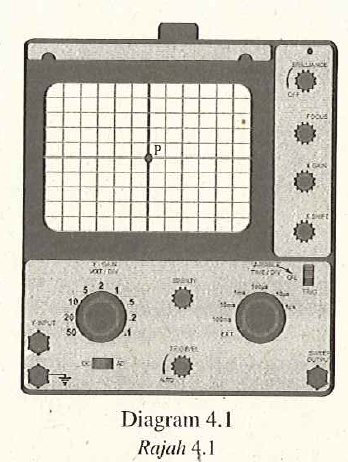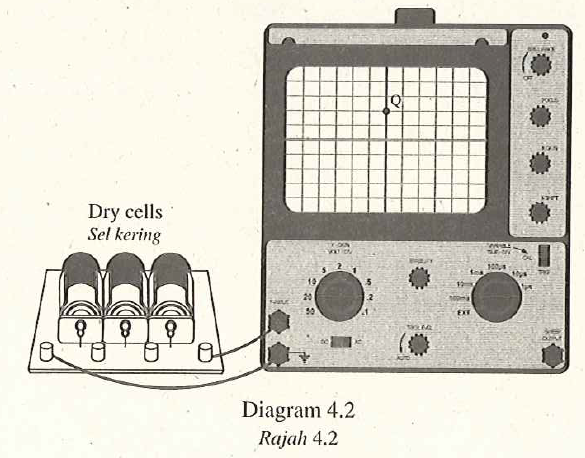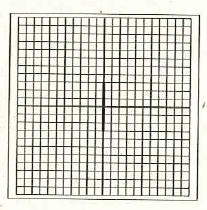# Structured Questions – Cathode Ray Oscilloscope

Diagram $$4.1$$ shows a bright spot, P, formed on the screen of a cathode-ray oscilloscope, C.R.O. when it is switched on.1. What is the meaning of cathode ray? [1 mark]
High speed election beam.
2. Explain how the bright spot is formed on the screen of the C.R.O. [2marks]
When the electrons hit the fluorescent screen, the screen glows and forms a light spot. The kinetic energy of the electrons changes to light energy.
3. Diagram $$4.2$$ shows the bright spot is displaced to $$Q$$ when a direct current, d.c. supply, is connected to the Y-input of the C.R.O.The Y-gain of the C.R.O is set at $$2 V /$$ division. Calculate the input voltage of the d.c. supply. [2 marks]

Output voltage
\begin{aligned} &=2 V +2 V \\ &=4 V \end{aligned}

1. Diagram $$4.3$$ shows an alternating current, a.c. supply, is connected to the C.R.O. The Y-gain of the C.R.O. remains at $$2 V /$$ division.Draw the output signal on the screen in Diagram $$4.3$$ when the peak voltage of the a.c. supply is $$6 V$$ and the time base is switched off. [2 marks]